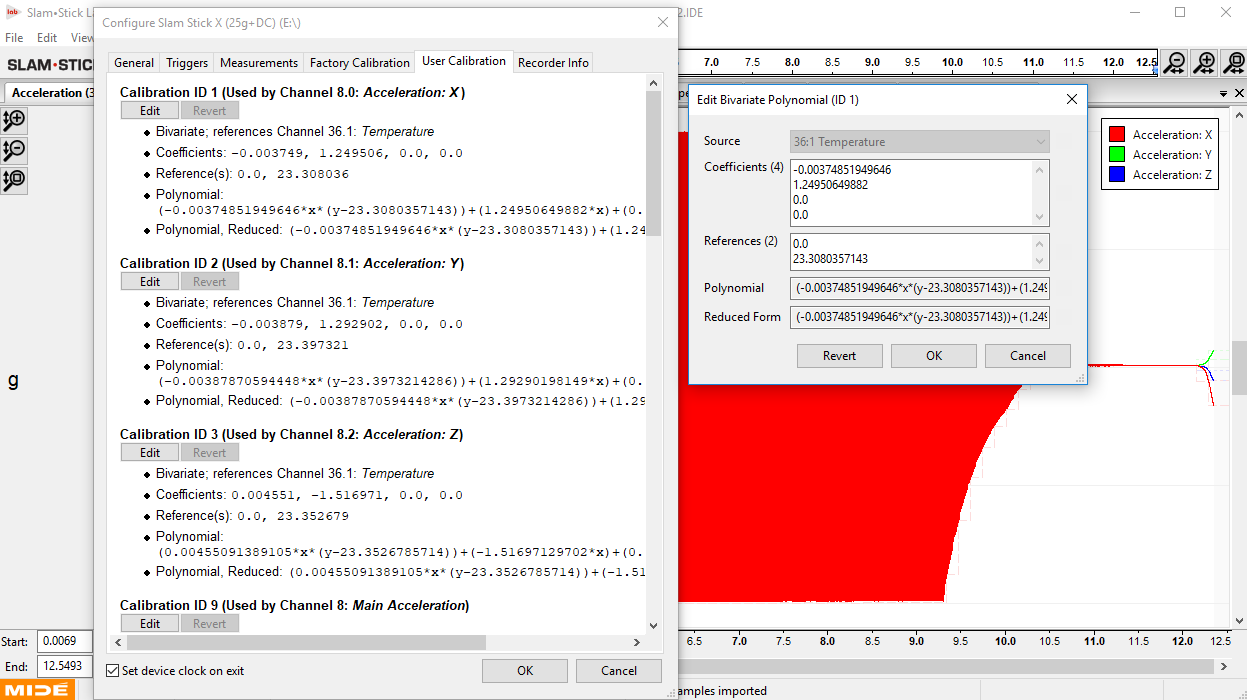# User Calibration Configuration

All enDAQ recorders are calibrated before leaving the facility, but this calibration is fully exposed to the user and can be adjusted.

# Factory Calibration

All enDAQ recorders are put through a calibration procedure that vibrates the device at 10g peak, 100 Hz in all three axes. A closed-loop shaker control system with a calibrated reference accelerometer is used to ensure the profile is as expected. Below is an image of one of these recordings/events, and here is the recording file.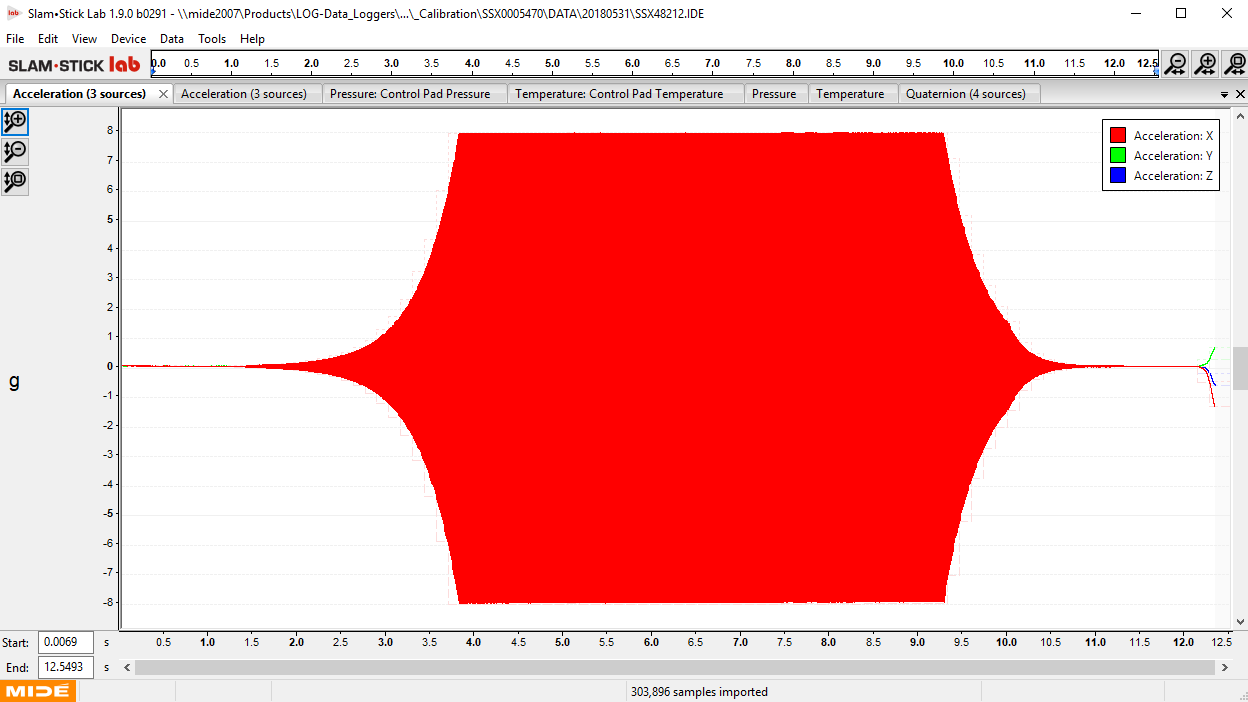As can be seen from the data, the recorder did not accurately measure the acceleration at 10g, instead, it was approximately 8g. A software script calculates this gain needed (1.25 = 10/8) and applies it to the device. In a similar calculation for DC response accelerometers, it calculates the DC offset to account for gravity. A calibration certificate ( download example here) is generated and saved to the device, an example is shown below.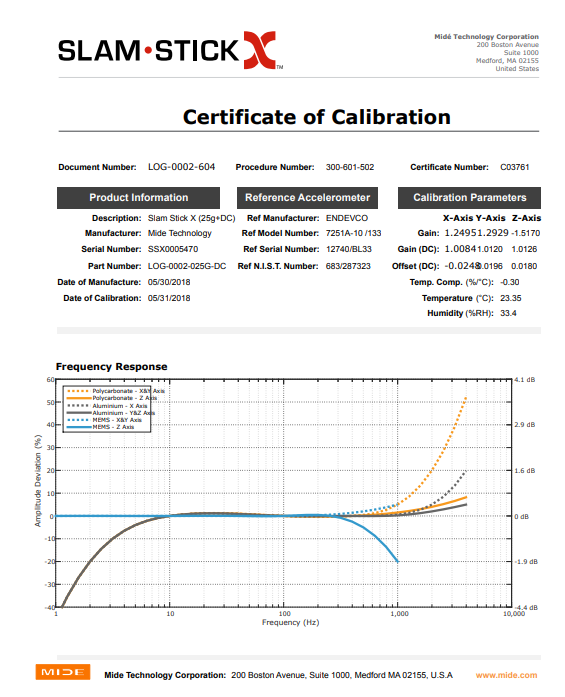The calibration applied to the recorder is also saved internally to the device, and these parameters can be accessed in the ‘Factory Calibration’ tab located next to the ‘Measurements’ tab in the configuration menu. This menu displays the specific gain and offset terms (and any bivariate terms if/when temperature compensation is needed) and it also displays the bivariate polynomials created from the initial calibration process of the enDAQ.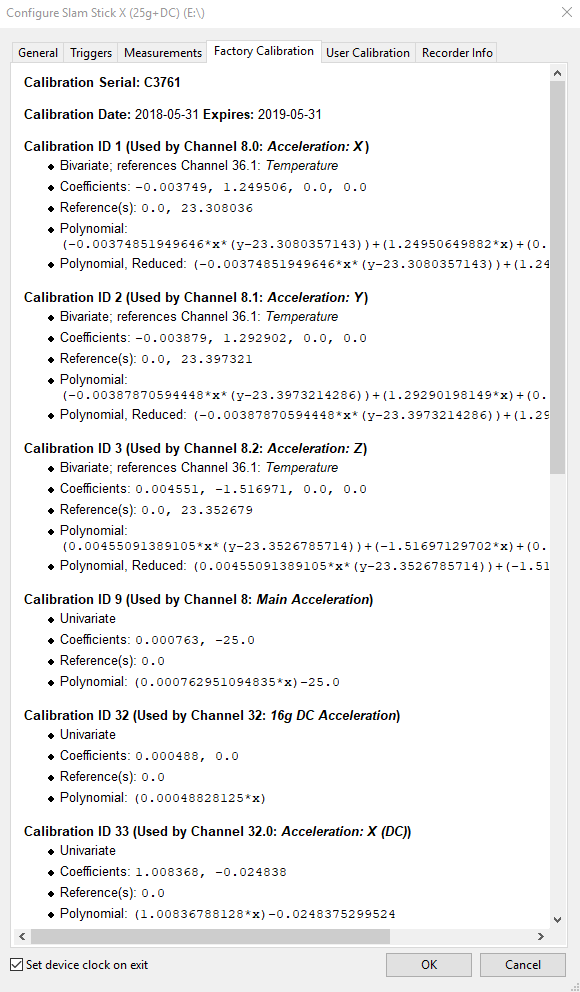These equations are how the enDAQ converts raw acceleration data into a calibrated form when displayed in the enDAQ Lab software. There are either one or two levels of calibration. One converts from raw integer data to engineering units and double format. The second level of calibration/conversion is applied by Mide to compensate for part-to-part variance. In the example above we have a 25g accelerometer. This means it can measure a 50g range and has 16-bit precision. The raw data is saved in 16-bit integer so in order to be converted to engineering units it needs to scale the 16-bit range to 50g. This is calculated by multiplying by 50 and dividing by 2^16 (65,536) which equals 0.00076 g. Then this range needs to be decreased to be centered around 0, so subtracting 1/2 the measurement range.

Then the per channel and part-to-part variance is calibrated with more simple terms that should be either close to 1 for gain, and close to 0 for compensating DC offset inaccuracies.

# Adjust Calibration Polynomial in Recorded Data

The calibration polynomial is applied to recorded data when plotting, analyzing, and exporting data. This calibration can be adjusted after the fact as necessary if/when it is determined that the calibration has drifted over time. To adjust this polynomial, navigate to the Data menu and 'Edit Calibration Polynomials...'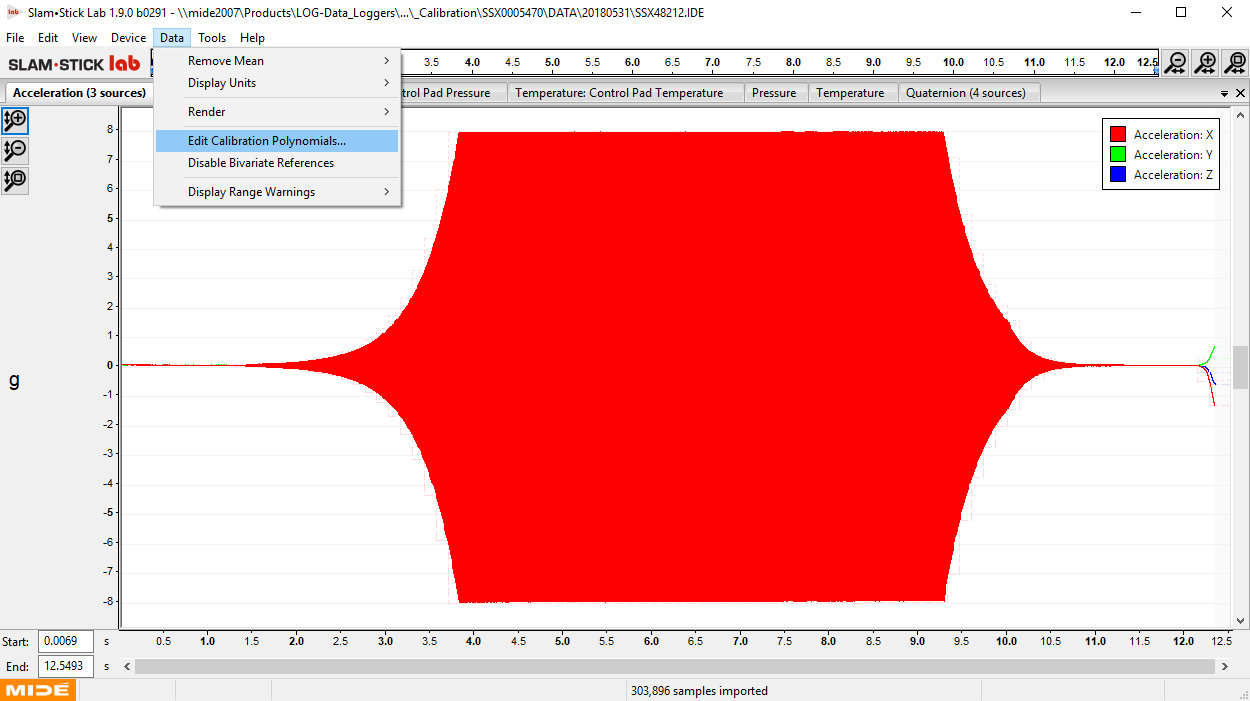In that menu, the calibration values can be adjusted to influence the plotted, analyzed or exported data. Back to the example of the recording during the calibration process, the calibration polynomials are all 1s and 0s. To compensate the data to reflect the required calibration terms, we can edit the gain term of the X axis to 1.25 which is determined by dividing the known reference acceleration (10g) by the value measured (8g) as shown.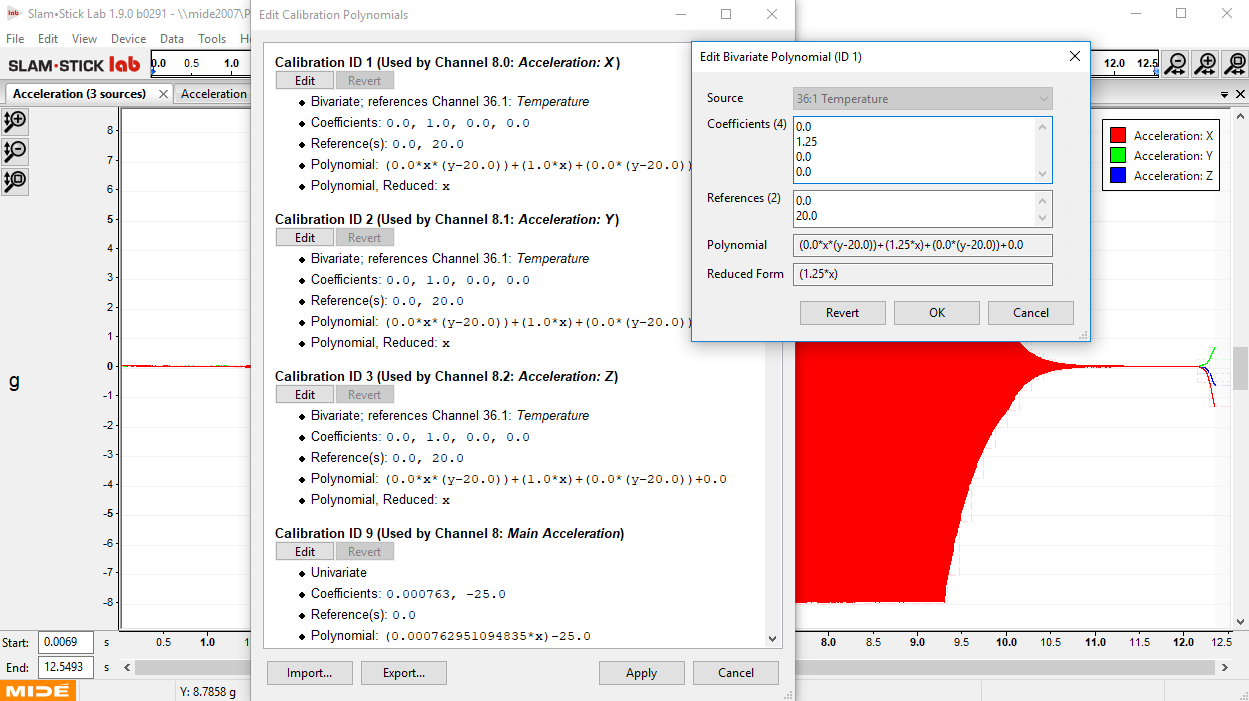Hitting apply will update the plotted data to reflect this change in the calibration polynomial as shown.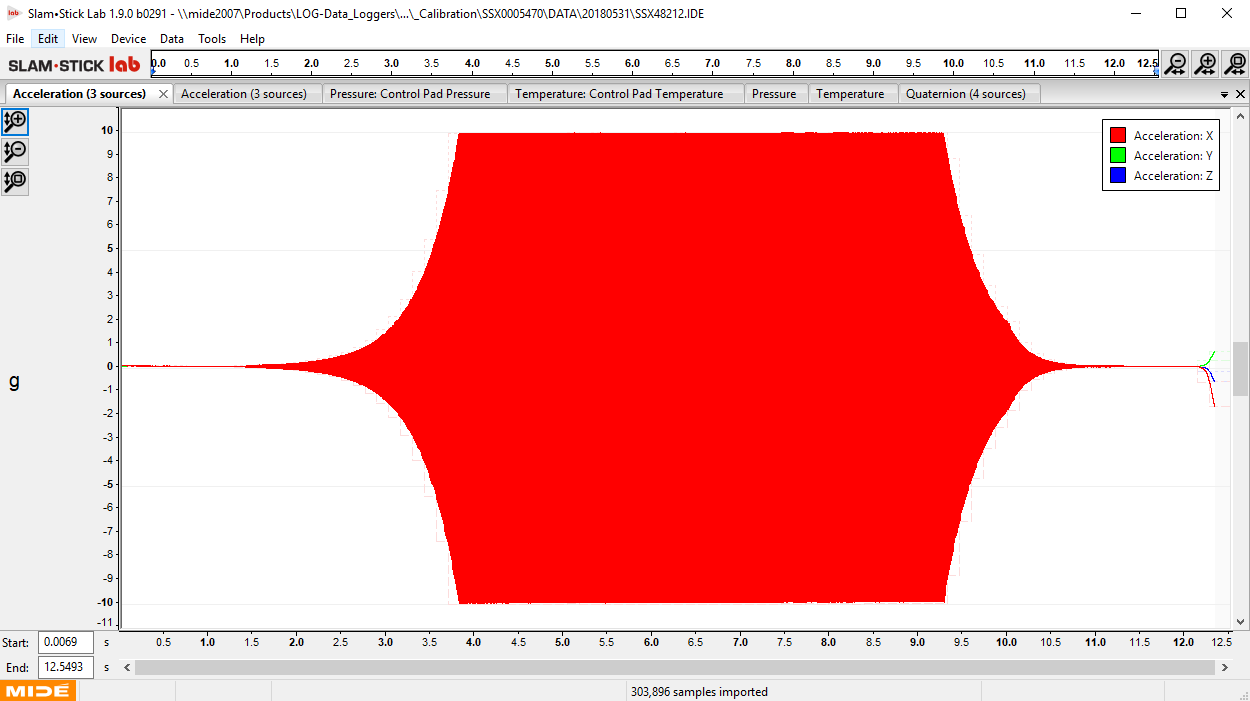The ‘Factory Calibration’ tab located next to the ‘Channels’ tab displays the bivariate polynomials created from the initial calibration process of the device. These equations are how raw acceleration data is converted into a calibrated form when displayed in the Lab software. These polynomials are also the required equations for exporting raw data into another analysis software, such as Python or MATLAB (See Exporting Data for more information on exporting data to MATLAB or Python).

# Apply a User Defined Calibration

If and when it is determined that the calibration on an enDAQ needs to be updated. The user has two options, first they can send it back to Mide for recalibration. Or second, they can apply their own calibration. To do so, navigate the configuration screen and the 'User Calibration' tab once an appropriate enDAQ has been identified.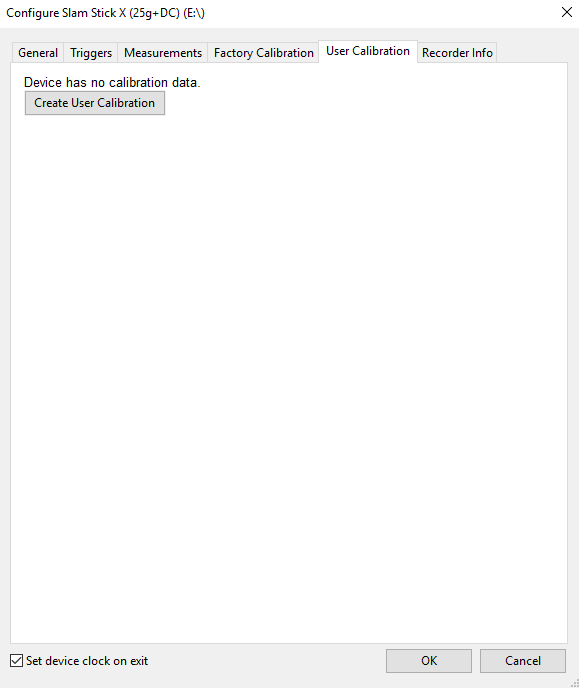1. In the event that an accelerometer offset has drifted away from its calibrated value, or the coefficients of the calibration polynomials saved to the device are inaccurate according to the actual device measurements, a user calibration can be performed to ensure the device continues recording accurately by using the Lab software.
2. To access the user calibration information, first, select the “Device” pulldown menu and select “Configure Device” just as you would to configure the sampling rate, set up a trigger, or view device information. Select the device you wish to adjust the calibration for, click “OK” and enter the configuration window as shown below.
3. Click the “Create User Calibration” button after selecting the “User Calibration” tab at the top of the configuration window. This will display the current calibration information for all channels of the device (Analog accelerometer: X, Y, Z; DC Accelerometer (If Applicable): X, Y, Z; Temperature; Pressure; ADC) and allows the user to edit each calibration value by clicking “Edit”. (See Below)
4. The important information to identify are the coefficients for each channel of the device. The first coefficient for the DC accelerometer (X, Y, Z channels) will correspond to the gain associated with the measurement in that channel. The second coefficient of the DC accelerometer channels refers to the offset detected by the device when no applied vibration is present and the accuracy of this offset can be determined by the user.
5. In order to verify that the coefficients are configured correctly for the DC accelerometer channels and the analog accelerometer channels, configure the sampling rate for the DC accelerometer to 3200 Hz (5000 Hz for the analog accelerometer) and place the enDAQ on a level surface (Z axis facing towards the ceiling); take a recording for 10 seconds without generating any external vibrations. Stop the recording and open the data file in the Lab. With the DC channel tab selected in the viewing window (The second Accelerometer tab), observe the value recorded for the X axis and Y axis in the data (i.e. the data should be a horizontal line with some scatter due to noise). This value is the active offset of the DC accelerometer and analog accelerometer in both the X and Y directions. The value measured should be on the order of ±0.01g for the DC accelerometer (will be larger for the analog accelerometer).
6. Confirm these values match the offset coefficients of the X and Y channels’ calibration information. If the recorded value deviates from the coefficient value by an order of magnitude or greater, click “Edit” as shown below.
7. The coefficients window should display the coefficients for the DC channel, the top value being the gain and the bottom value being the offset. If the offset value does not match the value measured from the recording by the margin mentioned above, delete the offset value displayed and enter the recorded value in its place.
8. The gain coefficient (i.e. the top coefficient in the window) should be within 5% of 1.0 (e.g. 0.95-1.05). If the gain coefficient is outside the mentioned range, set the gain coefficient to 1.
9. Click “OK” to finish editing the channel. Notice, the “Revert” button will now be active for the channels that were edited. If it is desired to return the calibration coefficients back to their original manufactured values, clicking the “Revert” button will do so. Click “Apply” when satisfied with the calibration coefficients entered.
10. To repeat this calibration for the Z axis, place the enDAQ on one of its sides on the flat surface (i.e. X or Y axis pointing up) and take another recording in the same manner as before. Check the data recording for the Z axis in the new data file and observe the DC offset. Adjust the Z axis polynomial coefficients in the same manner as the X and Y coefficients and click “Apply” to apply the correct coefficients.

This same process can be repeated for the piezoelectric accelerometer but this accelerometer has a dependence on the temperature channel. Therefore there are 4 coefficients, but only the first two are relevant. The first term is the temperature dependent gain term, this should equal -0.3% of the gain term, which is the second coefficient.

Please note that devices sent for recalibration with user calibration settings applied will have these settings disabled to allow for factory calibration. The user calibration can be restored by going into the DEVICE folder and renaming “usercal.dat~” to “usercal.dat”. This will remove the recent recalibration applied by enDAQ, but it will restore the user calibration. The updated calibration is always accessible under the Factory Calibration page.